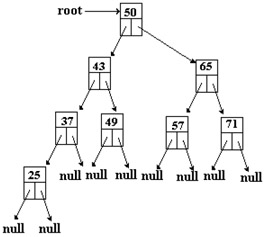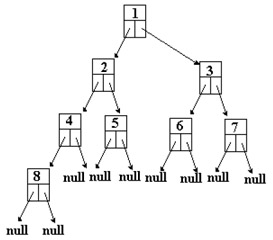# Binary Search Trees as Recursive Data Structures

In the discussion of the bst class in the last section, the class did not have all of the member functions required for the Basic Operation of an ADT Binary Search Tree. We still need two main member functions to be added to the class. First we need a member function display( ) that traverses the tree and displays the value of data of each of the nodes from the lowest valued node to the node with the highest value. Second the class needs a member function delete_node( ) that removes a particular node from the tree. So far in the discussion of the bst class, the member functions have been defined in an iterative manner. In this section the two new member functions will be defined recursively.

To define the function display( ) we want to start with the node that has the lowest data value. This node happens to be a leaf on the left side of the tree. Therefore we need to start at the root and move down the left branch of the root. After we have completed displaying the left branch of the root, the function would then display the value of data for the root. Next the function would proceed in a manner similar to the left branch and this time move down the right branch displaying the value of each data for these nodes. This sounds like a recursive process doesn't it!

For example suppose the member function display( ) is to act on the following binary tree. Recall that the pointer root of the bst object points to the binode whose data value is 50.The desire is to display the data value of the binode pointed to by the root of this tree and all of the data values below it. To achieve this, the function first needs to display the data in the left branch of the root, then display the data of the root and finally to display the data of each binode in the right branch. What we should see would be: 25, 37, 43, 49, 50, 57, 65, 71. To display the data values at each binode in the tree, we would display those of the branch to the left of the binode followed by displaying the data of the root binode and finally display the data of each binode in the right branch.

The function display( ) will have two parts, one part will contain the function traverse( ) that moves throughout the tree and the other part will display the data. To output to the screen, the function display( ) must use as an argument an object out of the ostream class. The function display( ) should be something like the following:` template<typename datatype> void bst<datatype>::display<datatype>(ostream & out) {    traverse(out,root); } template<typename datatype> void bst<datatype>::traverse(ostream out,binode * ptr) {   if(ptr != NULL)   {      traverse(out, ptr->left);      out << ptr->data << " ";      traverse(out,ptr->right);   } } `Notice in the body of the function traverse( ), that when a node of the tree was reached, the left branch was traversed first and then the node's data was displayed and finally the right branch was traversed. All of this was done in a recursive manner. To see how these functions work, see example: bst2.cpp and the header bst2h. This example uses Date objects from the header: date.h.

Now we need to consider how to delete a node using the member function delete_node(). In considering this problem we can see that there are three cases. The first type of node to be considered is a node with no children (it is a leaf) like the node with data value 8 as in following graphic:Second case would be the node that has only one child like the node above with data value 4. The third type of node to consider would have two children like the node above that has data value 2.

Suppose that we wanted to delete a node with the data value A. To begin the process we must first determine if the node exists. This will be done with the member function search( ) similar to the one used in bst.h above. The only modification will be the addition of a fourth argument parent that permits access to the parent of the node if the node is found. The function is the following:` template<typename datatype> bool bst<datatype>::search(datatype &item,binode* &location, binode* &parent) {   binode* ptr = root;   parent = NULL;   bool found = false;   for(;;)   {     if(found || (ptr == NULL))        break;     if(item < ptr->data)     {        parent = ptr;        ptr = ptr->left;     }     else        if(item > ptr->data)        {           parent = ptr;           ptr = ptr->right;        }        else        {           found = true;           location = ptr;        }   }   return found; } `In the first case, the node should be deleted and its parent's pointer pointing to it should be set to NULL. In the second case, the program must traverse to the child first and process it before the parent can be deleted. In the third case both children must be handled in the same way. The third case is the most difficult.` void bst<datatype>::delete_node(datatype& item) {   bool found;   binode* ptr,          *location,          *parent;   found = search(item,location,parent);   if(!found)      cerr << "Item not in the BST\n";   else   {      ptr = location;      if((ptr->left != NULL) && (ptr->right != NULL))      {          binode *successor = ptr->right;          parent = ptr;          while(successor->left != NULL)          {            parent = successor;            successor = successor -> left;          }          ptr->data = successor->data;;          ptr = successor;      }      binode * subtree = ptr->left;      if(subtree==NULL)         subtree = ptr->right;         if(parent==NULL)            root = subtree;         else            if(parent->left == ptr)               parent->left = subtree;            else               parent->right = subtree;      delete ptr;   } } `To see how a node could be deleted from a BST see example: bst3.cpp and the header bst3.h. This example uses Date objects from the header: date.h.

For the final example in this lecture, I have created a menued program that permits the testing of each of the individual member functions of the class bst. In addition it permits the input of the data from a disk file into the BST and then it sends the data to the binary file as the data is removed from the BST. For this example see bst4.cpp and the headers: bst4.h and date.h.